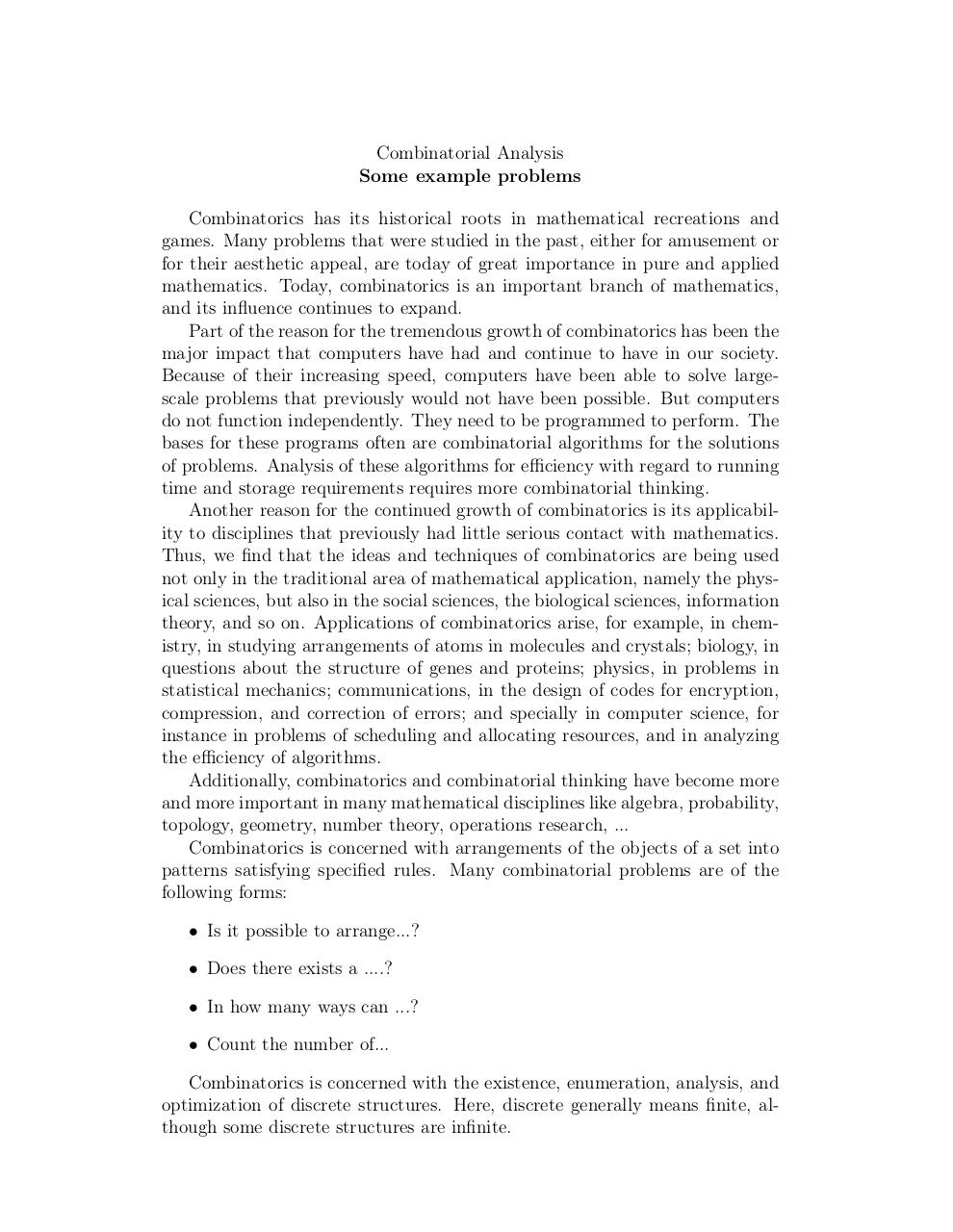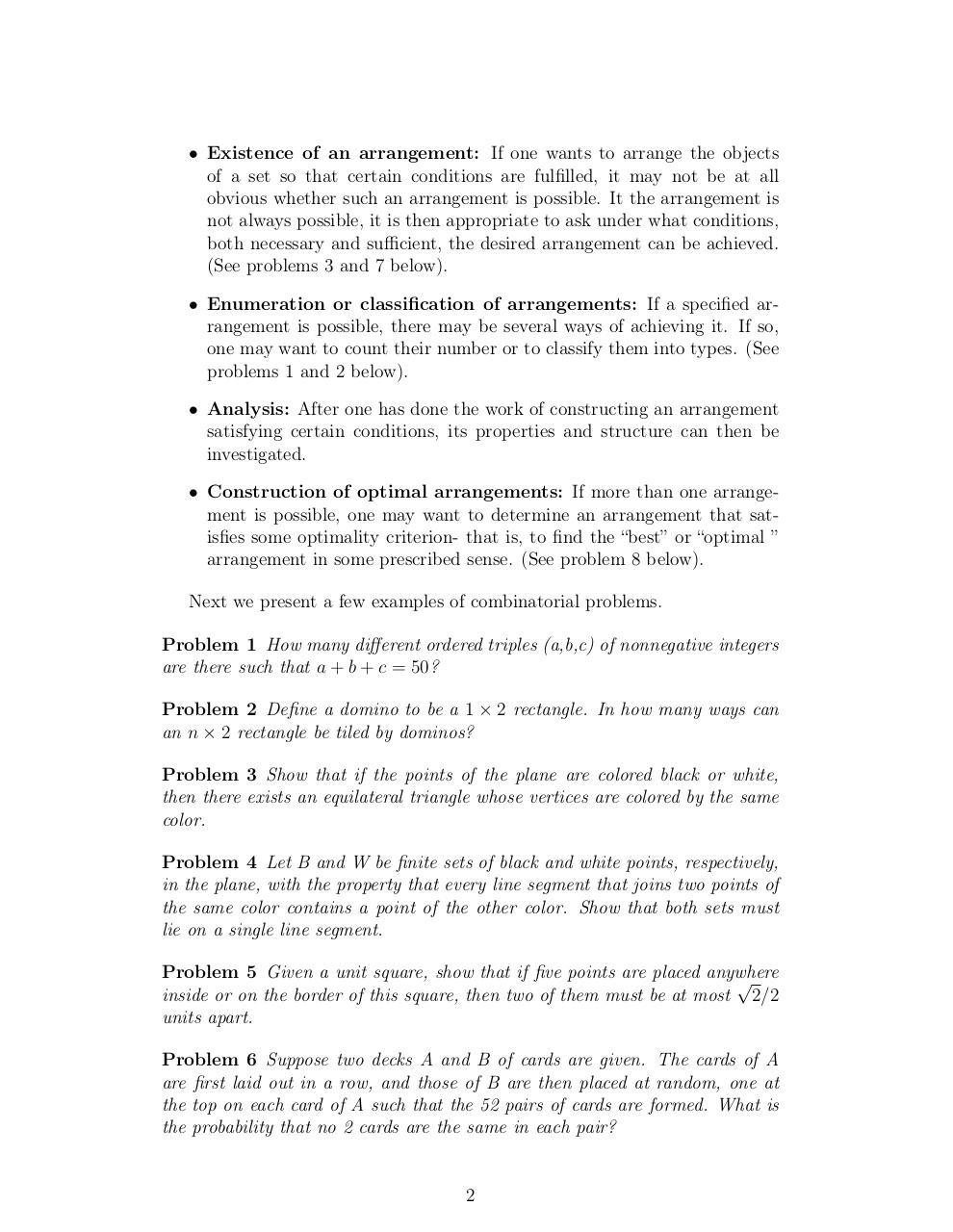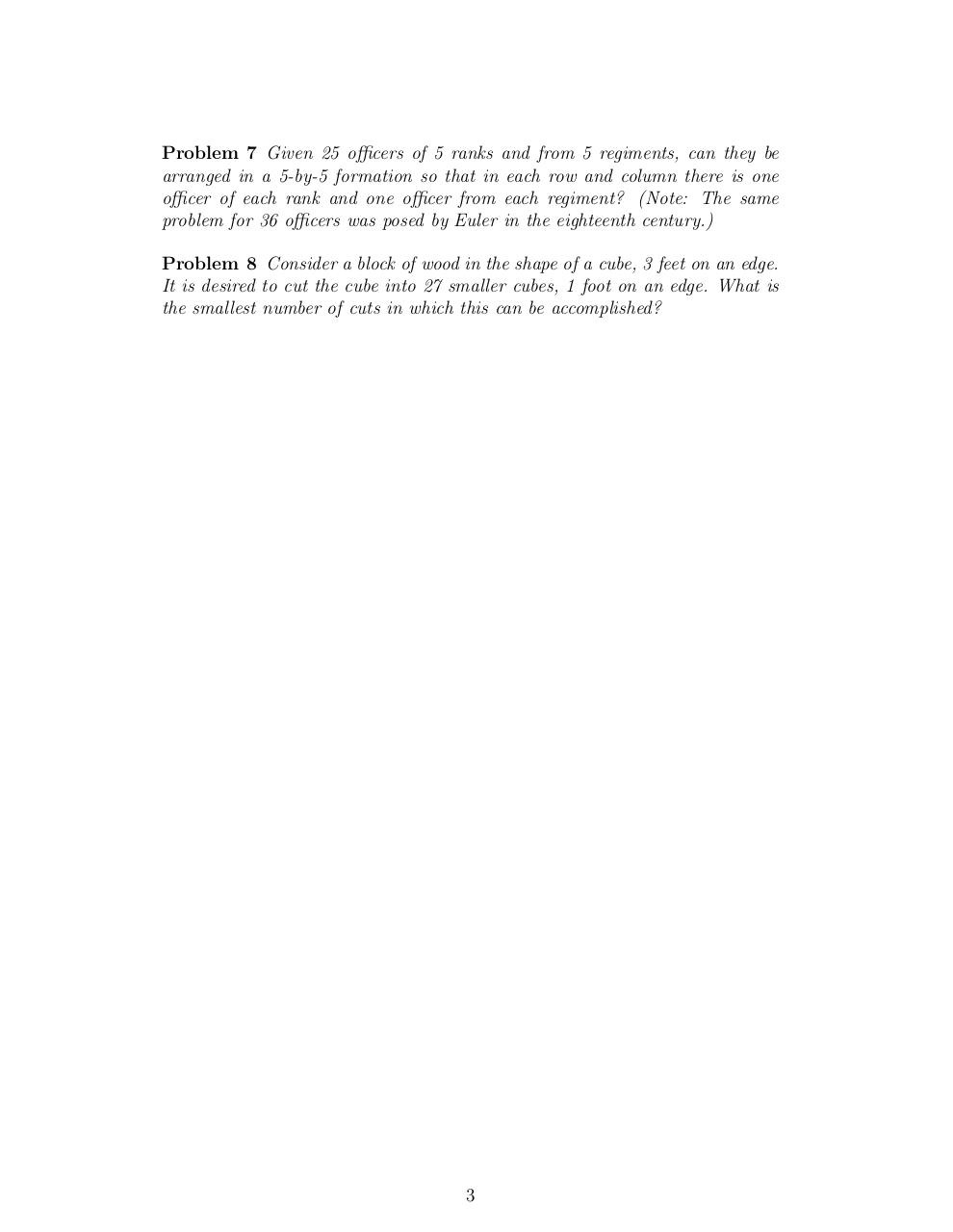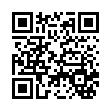# Day 1 Sample problems .pdf

### File information

Original filename: Day 1- Sample problems.pdf

This PDF 1.4 document has been generated by TeX / pdfTeX-1.40.3, and has been sent on pdf-archive.com on 24/01/2014 at 06:06, from IP address 169.231.x.x. The current document download page has been viewed 802 times.
File size: 87 KB (3 pages).
Privacy: public file

Day 1- Sample problems.pdf (PDF, 87 KB)

### Document preview

Combinatorial Analysis
Some example problems
Combinatorics has its historical roots in mathematical recreations and
games. Many problems that were studied in the past, either for amusement or
for their aesthetic appeal, are today of great importance in pure and applied
mathematics. Today, combinatorics is an important branch of mathematics,
and its influence continues to expand.
Part of the reason for the tremendous growth of combinatorics has been the
major impact that computers have had and continue to have in our society.
Because of their increasing speed, computers have been able to solve largescale problems that previously would not have been possible. But computers
do not function independently. They need to be programmed to perform. The
bases for these programs often are combinatorial algorithms for the solutions
of problems. Analysis of these algorithms for efficiency with regard to running
time and storage requirements requires more combinatorial thinking.
Another reason for the continued growth of combinatorics is its applicability to disciplines that previously had little serious contact with mathematics.
Thus, we find that the ideas and techniques of combinatorics are being used
not only in the traditional area of mathematical application, namely the physical sciences, but also in the social sciences, the biological sciences, information
theory, and so on. Applications of combinatorics arise, for example, in chemistry, in studying arrangements of atoms in molecules and crystals; biology, in
questions about the structure of genes and proteins; physics, in problems in
statistical mechanics; communications, in the design of codes for encryption,
compression, and correction of errors; and specially in computer science, for
instance in problems of scheduling and allocating resources, and in analyzing
the efficiency of algorithms.
Additionally, combinatorics and combinatorial thinking have become more
and more important in many mathematical disciplines like algebra, probability,
topology, geometry, number theory, operations research, ...
Combinatorics is concerned with arrangements of the objects of a set into
patterns satisfying specified rules. Many combinatorial problems are of the
following forms:
• Is it possible to arrange...?
• Does there exists a ....?
• In how many ways can ...?
• Count the number of...
Combinatorics is concerned with the existence, enumeration, analysis, and
optimization of discrete structures. Here, discrete generally means finite, although some discrete structures are infinite.

• Existence of an arrangement: If one wants to arrange the objects
of a set so that certain conditions are fulfilled, it may not be at all
obvious whether such an arrangement is possible. It the arrangement is
not always possible, it is then appropriate to ask under what conditions,
both necessary and sufficient, the desired arrangement can be achieved.
(See problems 3 and 7 below).
• Enumeration or classification of arrangements: If a specified arrangement is possible, there may be several ways of achieving it. If so,
one may want to count their number or to classify them into types. (See
problems 1 and 2 below).
• Analysis: After one has done the work of constructing an arrangement
satisfying certain conditions, its properties and structure can then be
investigated.
• Construction of optimal arrangements: If more than one arrangement is possible, one may want to determine an arrangement that satisfies some optimality criterion- that is, to find the “best” or “optimal ”
arrangement in some prescribed sense. (See problem 8 below).
Next we present a few examples of combinatorial problems.
Problem 1 How many different ordered triples (a,b,c) of nonnegative integers
are there such that a + b + c = 50?
Problem 2 Define a domino to be a 1 × 2 rectangle. In how many ways can
an n × 2 rectangle be tiled by dominos?
Problem 3 Show that if the points of the plane are colored black or white,
then there exists an equilateral triangle whose vertices are colored by the same
color.
Problem 4 Let B and W be finite sets of black and white points, respectively,
in the plane, with the property that every line segment that joins two points of
the same color contains a point of the other color. Show that both sets must
lie on a single line segment.
Problem 5 Given a unit square, show that if five points are placed anywhere

inside or on the border of this square, then two of them must be at most 2/2
units apart.
Problem 6 Suppose two decks A and B of cards are given. The cards of A
are first laid out in a row, and those of B are then placed at random, one at
the top on each card of A such that the 52 pairs of cards are formed. What is
the probability that no 2 cards are the same in each pair?

2

Problem 7 Given 25 officers of 5 ranks and from 5 regiments, can they be
arranged in a 5-by-5 formation so that in each row and column there is one
officer of each rank and one officer from each regiment? (Note: The same
problem for 36 officers was posed by Euler in the eighteenth century.)
Problem 8 Consider a block of wood in the shape of a cube, 3 feet on an edge.
It is desired to cut the cube into 27 smaller cubes, 1 foot on an edge. What is
the smallest number of cuts in which this can be accomplished?

3#### HTML Code

Copy the following HTML code to share your document on a Website or Blog

#### QR Code### Related keywords